# Mixed Review Math Worksheets

#### How to Create Sum and Difference Problems to Match a Value

Seeing how to ascertain the whole or distinction of numbers establishes some more elevated level issues and significant expertise in itself. At the point when these numbers are added together, the subsequent answer is known as the aggregate. When a number is deducted from another number, the outcome is known as the distinction. Follow the request for tasks and start by finishing any math situated in enclosures. For instance, if the numerical statement is 2 + 2(4-1), first take away one from 4. Utilize a mini-computer or do the computations in your mind or on paper. Increase and separation any numbers that require it from left to right. The numbers inside the brackets increase any numbers preceding an enclosure. Add and take away the excess numbers in the numerical question. The whole will be the consequence of adding numbers, while the distinction will be the aftereffect of taking them away. For example, in the numerical statement 4 + 3 - 5, the amount of 4 and 3 will be 7, and the contrast somewhere in the range of 7 and 5 will be 2. In this model, 2 is the last response to the numerical question.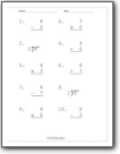###### Single Digit Operations Review

A nice way to review all your math facts.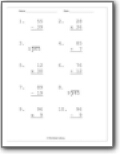###### Single and Double Digit Operations

Students will process the basic operators (add, subtract, multiply, and divide) between single and double digit numbers.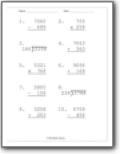###### Operations Between Three and Four Digit Numbers

We work along the same lines, but the values are much larger.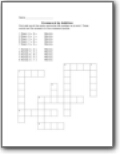First add up all the sums and write the number as a word. These words are the answers to the crossword puzzle.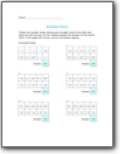###### Decimal Daze

Follow the number maze making only straight lines to the right and down so that the sum of the numbers equals the answer to the puzzle. Start in the upper left corner, end at the Answer square.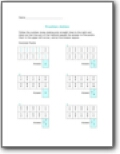###### Fraction Action

Follow the number maze making only straight lines to the right and down so that the sum of the fractions equals the answer to the puzzle. Start in the upper left corner, end at the Answer square.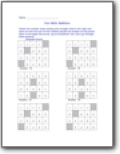Follow the number maze making only straight lines to the right and down so that the sum of the numbers equals the answer to the puzzle. Start in the upper left corner, go to the bottom line. Don’t go through blank squares.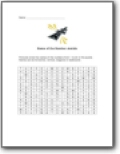###### Backwards Name of the Number Puzzle

Find and circle the names of the numbers from 1 to 20 in the puzzle. All the names of the numbers are spelled backwards. Number names can be horizontal, vertical or diagonal. A sample answer is shown in red. The names of five geometric shapes are also hidden in the puzzle. These are spelled either forward or backward and can be horizontal or vertical but not diagonal.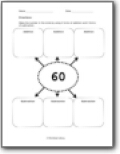###### Create Your Own Sums and Differences

Make the number in the circle by using 3 forms of addition and 3 forms of subtraction. As you will see below we have a ton of these sheets and they come in a ten-pack the end results are listed below.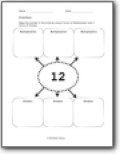###### Multiplication and Division Version

Make the number in the circle by using 3 forms of Multiplication and 3 forms of Division. The values are mixed and not sequential.